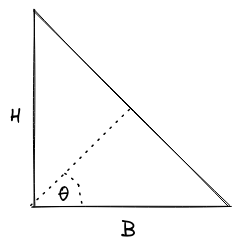# Hasinur Has Twomoshiur_cse15RUET CodeSmash 2018
Limits 1s, 512 MB

You all have heard the name of great landlord Hasinur the Generalman. He has a huge land. The land has a shape of a right triangle with base B and height H. Hasinur the Generalman wants his land to be divided between his two wives. The distribution process is to cut the triangle from the intersecting point of base and height to the hypotenuse of the triangle with an angle of 𝛳 with the base. You can illustrate the distribution process in the following figure.Now his younger wife has hired you to check how much land (area of land) she and other wife will receive if the distribution process is performed by Hasinur the Generalman. The lower part of the distribution line will be received by the younger wife and the other part by the elder wife.

## Input

First line will have an integer $T$ ($T ≤ 1000$), the number of test cases. Then the following $T$ line have three integers each $B$, $H$ ($1 ≤ B, H ≤ 1000$) and $\theta$ ($0 < \theta < 90$) as described above.

## Output

Print "Case x: " (w/o quotes) where $x$ is the case number, and then two space-separated floating point number, exactly three digits after the decimal point, first one is the land that will be received by the younger wife and then by the elder one.

## Sample

InputOutput
1
4 4 45
Case 1: 4.000 4.000


In case you need to use $\pi$, value of $\pi = acos(-1.00)$, basically $cos^{-1}$.

In C or C++, writing the code double PI = acos(-1.00); would work.

### Submit